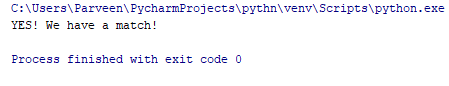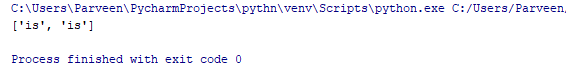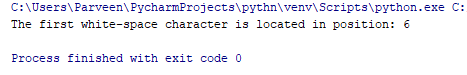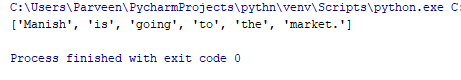×

### Html & Html5

Introduction to Html

Component of Html

Html Form

Html5 basics

Html5 Grid

Html5 Graphics

Html5 Media

Html5 APIs

### Python and Pandas

Introduction to Python

Component of Python

### Python RegEx

RegEx stands for regular expression and it is the sequence of characters that forms a search pattern means we can use it for checking if the particular string contains the specified search pattern.
Let's understand this by the following program :-

import re

txt = "Manish lives in India"

x = re.search("^Manish.*India\$", txt)

if (x):

print("YES! We have a match!")

else:

print("No match")

### Output of this ProgramSo we can see our both “Manish” and “India” are in the string and it tells us the correct output.

We are having other set of functions too which allows us to search a string , which are describe as follows:-

Function Description
findall Returns a list containing all matches
search Returns a math object if there is a match anywhere in the string
split Returns a list where the string has been split at each match
sub Replaces one or many matches with a string

findall() function

As we have discussed above also that the findall() function use to return a list containing all matches.

For ex:- Here in the below program we can find the occurrence of “es”

import re

str = "Manish is going to the market."

x = re.findall("is",str)

print(x)

### Output of this ProgramHere it tells us that two times the “is” word is there in the string.

search() function

This function is use to search the string for a match. Let’s understand it by the following program :-

import re

str = "Manish is going to the market."

x = re.search("\s",str)

print("The first white-space character is located in position:", x.start())

### Output of this ProgramSo here like this we can search whatever we like in our string.

split function()

As we have discussed above also that split function use to split all the string characters.

import re

str = "Manish is going to the market."

x = re.split("\s",str)

print(x)

### Output of this ProgramHere we can see whole string is splitted.

sub() function

As we know sub function() is use to replace one or many matches with a string.

Let’s understand this by the following program :-

import re

str = "Manish is going to the market."

x = re.sub("\s", "8",str)

print(x)

### Output of this ProgramSo like this we can replace.

python training insitute| Best IT Training classes in Gurgaon | python training